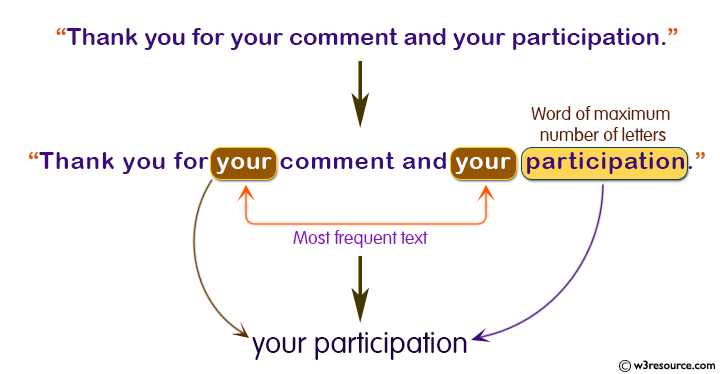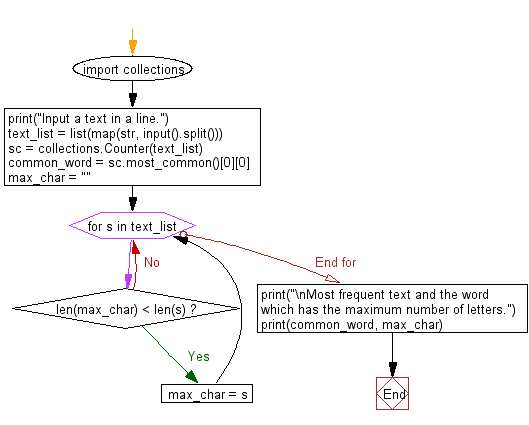﻿ Python: Reads a text and prints two words - w3resource# Python: Reads a text and prints two word

## Python Basic - 1: Exercise-47 with Solution

Write a Python program which reads a text (only alphabetical characters and spaces.) and prints two words. The first one is the word which is arise most frequently in the text. The second one is the word which has the maximum number of letters.

Note: A word is a sequence of letters which is separated by the spaces.

Input:
A text is given in a line with following condition:
a. The number of letters in the text is less than or equal to 1000.
b. The number of letters in a word is less than or equal to 32.
c. There is only one word which is arise most frequently in given text.
d. There is only one word which has the maximum number of letters in given text.

Input:

Pictorial Presentation:Sample Solution:

Python Code:

``````import collections
print("Input a text in a line.")
text_list = list(map(str, input().split()))
sc = collections.Counter(text_list)
common_word = sc.most_common()
max_char = ""
for s in text_list:
if len(max_char) < len(s):
max_char = s
print("\nMost frequent text and the word which has the maximum number of letters.")
print(common_word, max_char)
``````

Sample Output:

```Input a text in a line.

Most frequent text and the word which has the maximum number of letters.
```

Flowchart:Python Code Editor:

Have another way to solve this solution? Contribute your code (and comments) through Disqus.

What is the difficulty level of this exercise?

Test your Programming skills with w3resource's quiz.

﻿

## Python: Tips of the Day

Iterating over dictionaries using 'for' loops:

I am a bit puzzled by the following code: d = {'x': 1, 'y': 2, 'z': 3} for key in d: print key, 'corresponds to', d[key] What I don't understand is the key portion. How does Python recognize ...

key is just a variable name.

```for key in d:
```

For Python 3.x:

```for key, value in d.items():
```

For Python 2.x:

```for key, value in d.iteritems():
```

To test for yourself, change the word key to poop.

In Python 3.x, iteritems() was replaced with simply items(), which returns a set-like view backed by the dict, like iteritems() but even better. This is also available in 2.7 as viewitems().

The operation items() will work for both 2 and 3, but in 2 it will return a list of the dictionary's (key, value) pairs, which will not reflect changes to the dict that happen after the items() call. If you want the 2.x behavior in 3.x, you can call list(d.items()).

Ref: https://bit.ly/37dm0Qo